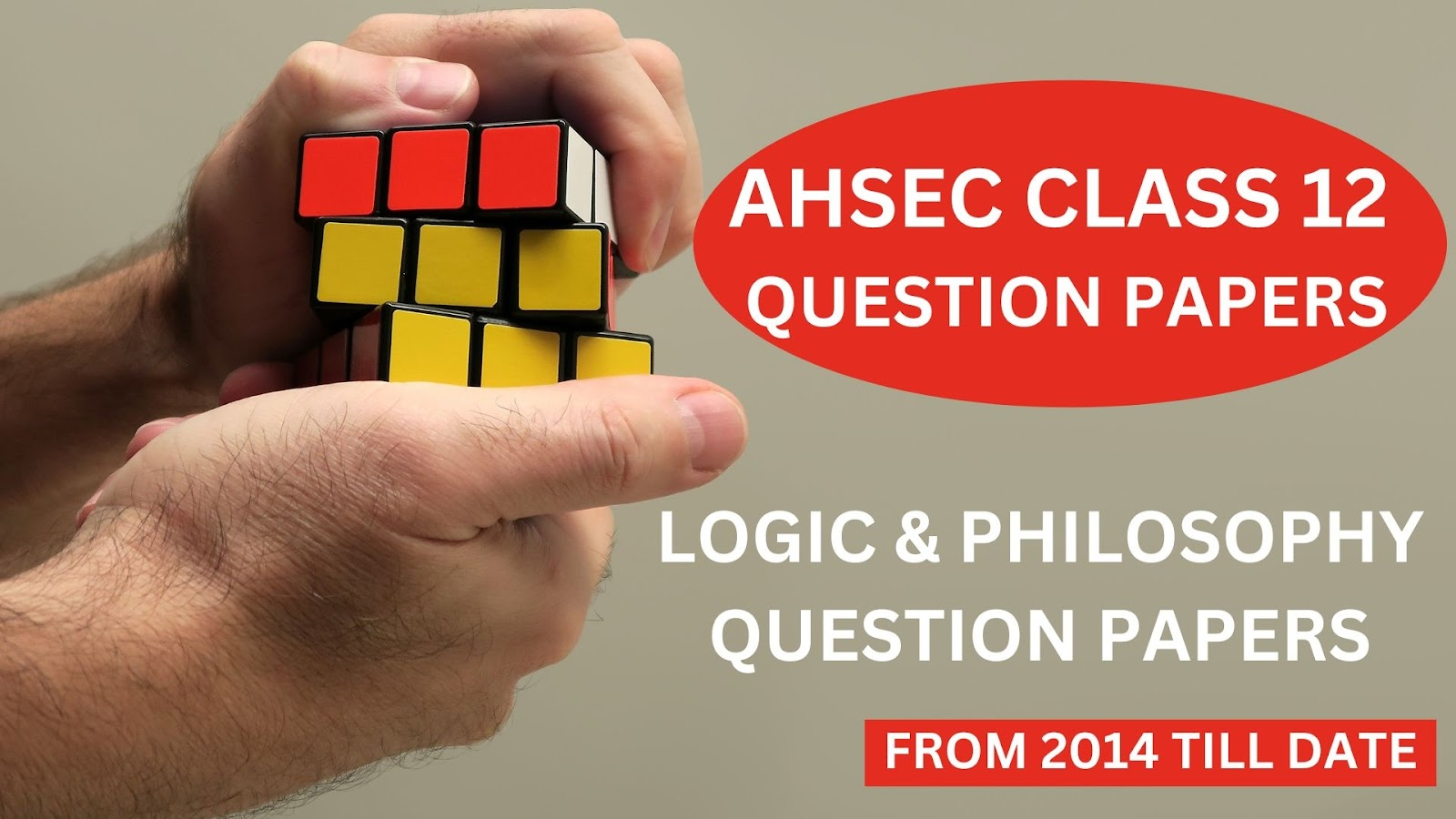# Logic and Philosophy Question Paper' 2015 [AHSEC Class 12 Question Papers]## Logic and Philosophy Question Paper' 2015AHSEC Class 12 Question PapersFull Marks: 100Pass Marks: 30Time: 3 hours

The figures in the margin indicate full marks for the questions
1. Give very short answer:                                           1x12=12
a)      “The premises of an inductive inference offer certainty for the truth of the conclusion”. – Is it true?
b)      Which of the following statements is true?
1)      The law of causation is a material ground of induction.
2)      Observation and the law of causation are the formal grounds of induction.
3)      Observation and experiment are the formal grounds of induction.
4)      None of the above statements is true.
c)       “Observation is finding a fact and experiment is making one.” – Who did say this statement?
d)      Which one of the following statement is incorrect?
1)      Observation is a perception with a definite purpose in view.
2)      Observation is a perception of natural events.
3)      Observation is not a perception of events under natural conditions.
4)      In observation, the natural events are not full under the control of observer.
e)      Which is the last stage of hypothesis suggested by Mill’s definition of hypothesis?
f)       What is the meaning of condition of ‘testability’ of valid hypothesis?
g)      “When heat is applied to ice, then ice melts. When heat is not applied to ice, then ice does not melt. Therefore, heat is the cause of the melting of ice.”
Name the experimental method employed in the above argument.
h)      “The possibility of the doctrine of the plurality of causes cannot frustrate the method of agreement.” – Is it correct?
i)        Who among the following philosophers is not an idealist?
1)      Berkeley.
2)      G. E. Moore.
j)        Name the philosopher associated with the statement – “Thought and Reality are at bottom identical”.
k)      What is the meaning of the Latin word ‘Religare’?
l)        Mention any one similarity between Religion and Morality.
2. State two points of difference between deduction and induction.                       1x2=2
3. Define bad analogy with suitable example.                                     1+1=2
4. State any two characteristics of analogy.                                          2
5. Define material cause with an example.                                           1+1=2
6. Briefly explain any one criticism against Mill’s paradox of induction.                     2
Or
Why Mill’s paradox of induction is said to commit the fallacy of petition principia or fallacy of arguing in a circle?       2
7. What do you mean by a working hypothesis?                                                2
8. Briefly explain any one condition of a valid hypothesis with the help of a suitable example.                     2
9. Why do some logicians regard the method of agreement as a method of single agreement?                   2
10. Give a concrete example of joint method of agreement and difference.                        2
11. What are primary qualities? Give example.                                   1+1=2
12. Briefly express any two characteristics of objective idealism.                                                2
Or
Briefly express any two points of criticism against objective idealism.                      2
13. State any two essential characteristics of religion.                                      2
14. What is induction? State two differences between deduction and induction.                                               2+2=4
15. “All inferences are analogical at bottom.” – Explain the significance of this remark.                    4
16. What is a condition? Briefly explain positive and negative condition with the help of suitable example.            1+3=4
17. What do you mean by fallacy of mal-observation? Distinguish between individual mal-observation and universal mal-observation with the help of suitable example.                           1+3=4
Or
Distinguish between fallacy of non-observation of essential instances and fallacy of non-observation of essential circumstances with the help of suitable example.                                             4
18. Distinguish between explanatory hypothesis and descriptive hypothesis.                      2+2=4
19. What is a crucial instance? Briefly explain a crucial instance obtained by experiment with the help of a suitable example.                                                 2+2=4
20. State two advantages and two disadvantages of the method of difference.                  2+2=4
Or
State two advantages and two disadvantages of the method of residues.                             2+2=4
21. What do you mean by naïve realism? State any two differences between naïve realism and scientific realism.                2+2=4
Or
What do you mean by naïve realism? Briefly express any two points of criticism against this type of realism.        2+2=4
22. Briefly express any four characteristics of naïve realism.                         4
Or
Briefly express any four characteristics of scientific realism.                         4
23. What is habitual action? Why is this type of action regarded as object of moral judgement?                  2+2=4
24. Define the method of agreement in your own words and give a concrete example of this method where we proceed from effect to cause. Why is this method called pre-eminently a method of observation?                                             2+2+2=6
Or
Define the method of residues in your own words. Why do some logicians regard this method as a method of deduction? Explain.                                                2+4=6
25. What is Berkeley’s subjective idealism? On what grounds Berkeley has said that primary and secondary qualities are inseparable?                                                      2+4=6
Or
Critically discuss objective idealism of Hegel.                                       6
26. Briefly discuss any three of the different elements involved in the mental stage of voluntary actions.     2x3=6
Or
What do you mean by non-moral actions? Briefly explain any five non-moral actions.      1+5=6
27. Critically explain any one of the following views:                                        6x1=6
a)      Religion is the source of morality.
b)      Morality is the source of religion.
c)       Religion and morality are independent of each other.

***